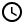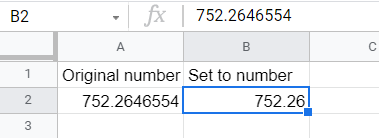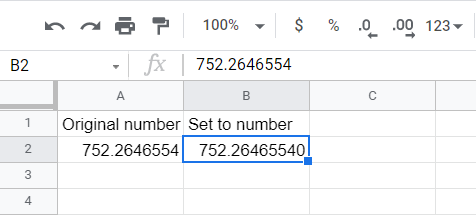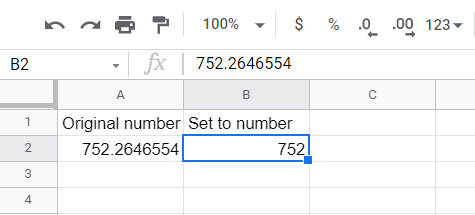# Increase or Decrease the Number of Decimals Places of Numbers in Google Sheets

Learn how to modify the decimal place of numbers in google sheets to the desired precision. This tool will give you greater control fo both data and display in spreadsheets.1. Increase the number of decimal places
2. Decrease the number of decimal placesWhen you enter a number in a cell or cells in Google Sheets that is formatted for a number, it rounds off the excess decimals to a set precision. By default, it is set to two decimal places:Original number with several decimal places rounded off to having only two decimal places.

You can increase or decrease the number of decimal places displayed by Google Sheets.

## Increase the number of decimal places displayed

To increase the number of decimal places displayed, select the cell or cells you want to change, then click the following icon in the toolbar above the sheet:

The result is:

If you try adding more decimal places than were originally included, zeros will be added:The number having more decimal places than the original number in the source cell has will have additional zeroes at the end of it.

## Decrease the number of decimal places

To decrease the number of decimal places displayed, select the cell or cells you want to change, then click the following icon in the toolbar above the sheet:

The function will no longer work when you have removed all the decimal places:The number has no more decimal places. The button doesn’t work when all the decimal places are removed.
Oops! Something went wrong while submitting the form.

August 4, 2021When you enter a number in a cell or cells in Google Sheets that is formatted for a number, it rounds off the excess decimals to a set precision. By default, it is set to two decimal places:Original number with several decimal places rounded off to having only two decimal places.

You can increase or decrease the number of decimal places displayed by Google Sheets.

## Increase the number of decimal places displayed

To increase the number of decimal places displayed, select the cell or cells you want to change, then click the following icon in the toolbar above the sheet:

The result is:

If you try adding more decimal places than were originally included, zeros will be added:The number having more decimal places than the original number in the source cell has will have additional zeroes at the end of it.

## Decrease the number of decimal places

To decrease the number of decimal places displayed, select the cell or cells you want to change, then click the following icon in the toolbar above the sheet: